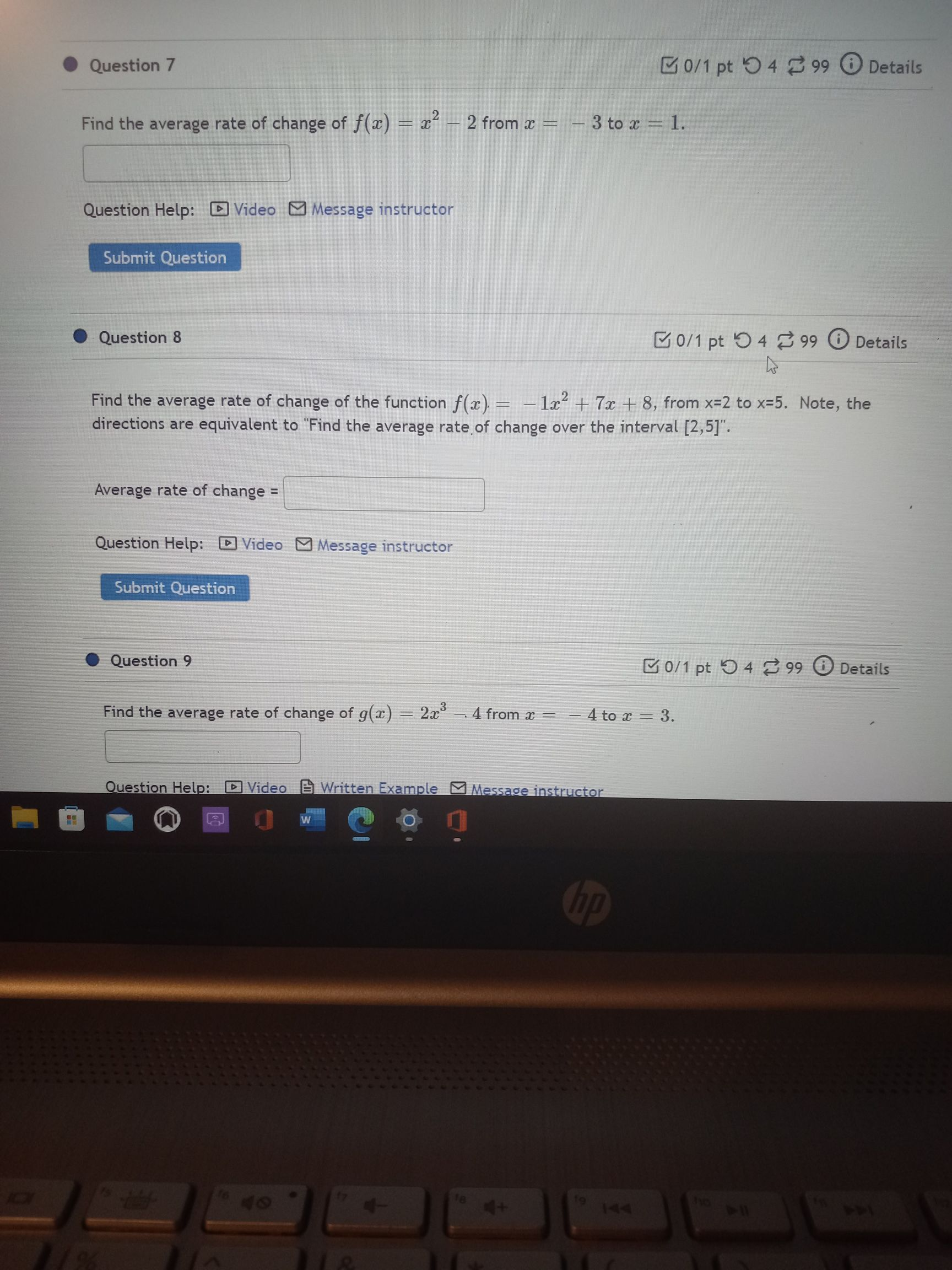### ¿Todavía tienes preguntas de matemáticas?

Pregunte a nuestros tutores expertos
Algebra
Pregunta7. Find the average rate of change of $$f ( x ) = x ^ { 2 } - 2$$ from $$x = - 3$$ to $$x = 1$$.

8. Find the average rate of change of the function $$f ( x ) = - 1 x ^ { 2 } + 7 x + 8 ,$$ from $$x = 2$$ to $$x = 5$$ . Note, the directions are equivalent to "Find the average rate of change over the interval $$[ 2,5 ]$$ ".

9. Find the average rate of change of $$g ( x ) = 2 x ^ { 3 } - 4$$ from $$x = - 4$$ to $$x = 3$$ .

9.$$\frac{34}{7}$$